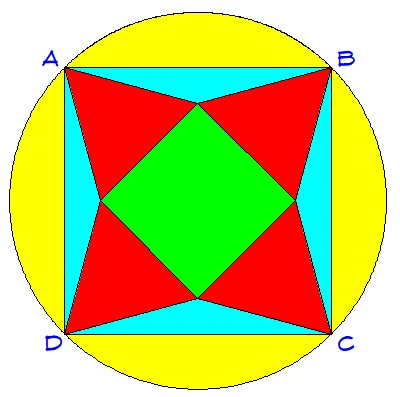The story of the green square.

Geometry Level 3The green square in the diagram is symmetrically placed at the center of the circle. Four red equilateral triangles are drawn such that square $ABCD$ is formed. Let $y,b,g,$ and $r$ be the areas of the yellow, blue, green, and red regions, respectively.

If $r=4\sqrt{3}$, find $y+g-b$.

×